Function Repository Resource:

# MedianQuantileAround

Calculate the median of a list along with its uncertainty

Contributed by: Ting Sun
 ResourceFunction["MedianQuantileAround"][{x1,x2,…}] gives an Around object whose value is the median of the xi and whose uncertainty is the interquartile range. ResourceFunction["MedianQuantileAround"][{x1,x2,…},δ] gives an Around object with uncertainty specified by δ. ResourceFunction["MedianQuantileAround"][{x1,x2,…},{δ-,δ+}] gives an Around object with upper and lower uncertainties specified by δ- and δ+.

## Details and Options

The "value" part of the Around object retured is calculated using the built-in Median and Quantile functions.
ResourceFunction["MedianQuantileAround"][{x1,x2,},δ] is defined to be {Quantile[{x1,},0.5+δ]-Median[{x1,}],Quantile[{x1,},0.5-δ]-Median[{x1,}]}.
ResourceFunction["MedianQuantileAround"][{x1,x2,},{δ-,δ+}] is defined to be {Quantile[{x1,},0.5-δ-]-Median[{x1,}],Quantile[{x1,},0.5+δ+]-Median[{x1,}]}.

## Examples

### Basic Examples (1)

Calculate the median of some numbers with uncertainty represented by interquartile range:

 In:=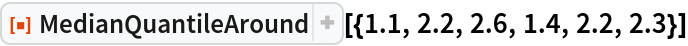Out=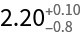### Scope (2)

The size of the inter-quantile range to use can be specified as a second argument:

 In:=Out=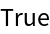MedianQuantileAround also works on quantities with units:

 In:=Out=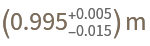### Possible Issues (1)

MedianQuantileAround does not work with symbolic expressions:

 In:=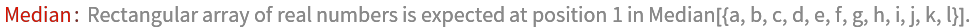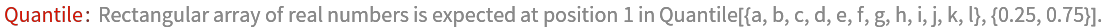Out=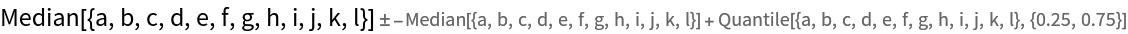Ting Sun

## Version History

• 1.0.0 – 17 April 2020